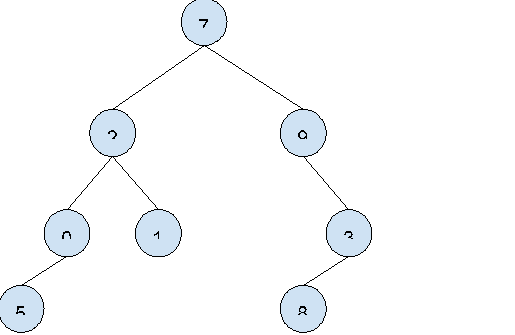# Even size subtree in n-ary tree in C++

In this problem, we are given an adjacency list that denotes an n-ary tree. Our task is to find the number of even size subtree in n-ary tree.

N-ary tree is defined as a collection of nodes normally represented hierarchically in the following manner.

The tree is started at the root node.

Each node of the tree maintains a list of pointers to its child nodes.

The number of child nodes is less than or equal to m.

## Let’s take an example to understand the problem,

Input:Output: 4

Explanation:

Tree rooted with 7 has an even size.
Tree rooted with 2 has an even size.
Tree rooted with 0 has an even size.
Tree rooted with 3 has an even size.

## Solution Approach −

A simple approach is by counting all child nodes for a given node, if it is even increase the evenTreeCount. For this we will use DFS, and find the length of SubTree for the given node.

We can do this using a single traversal on the tree. By recursively finding the size of subtrees of each child node and then check the size and if its is even, increase the evenTreeCount otherwise leave it.

## Example

Live Demo

#include <bits/stdc++.h>
using namespace std;

int countEventSizeSubTree(vector<int> adj[], int n, int v, int&amp; EvenCount){

int size = 1;
for (auto ele : adj[v]) {
size += countEventSizeSubTree(adj, n, ele, EvenCount);
}
if (size % 2 == 0)
EvenCount++;
return size;
}

int main(){

int n;
n = 10;

}
Even Size SubTree are 0